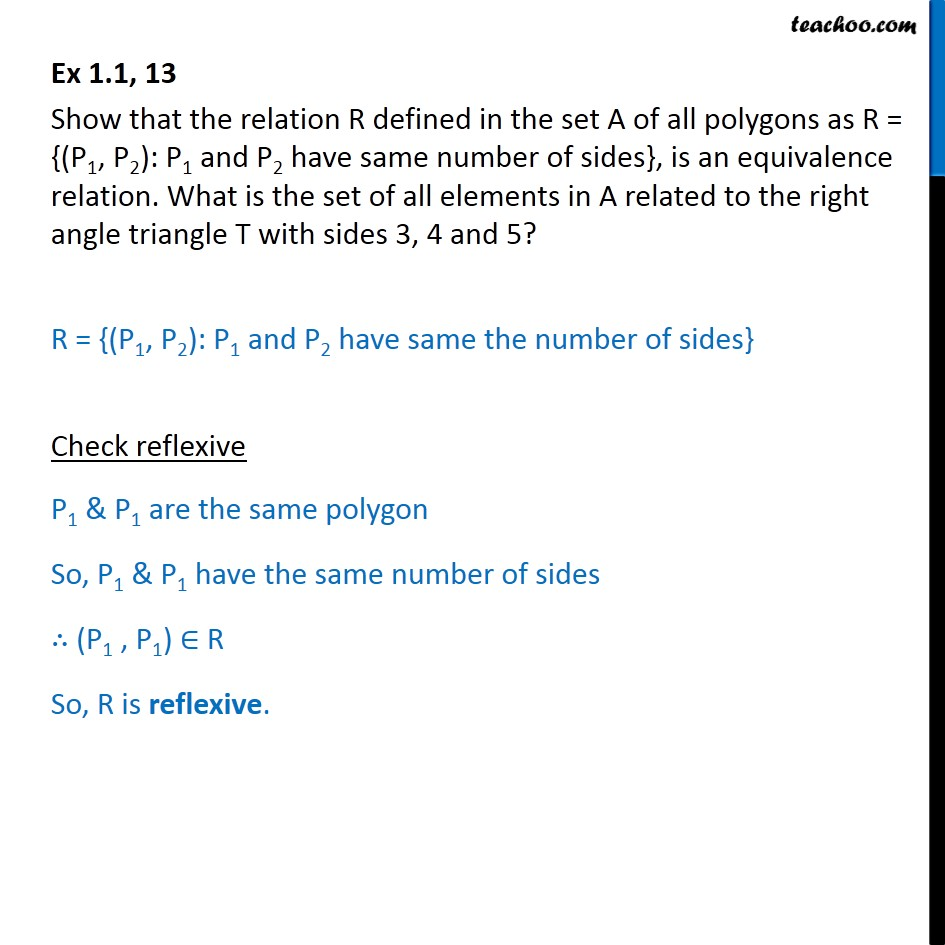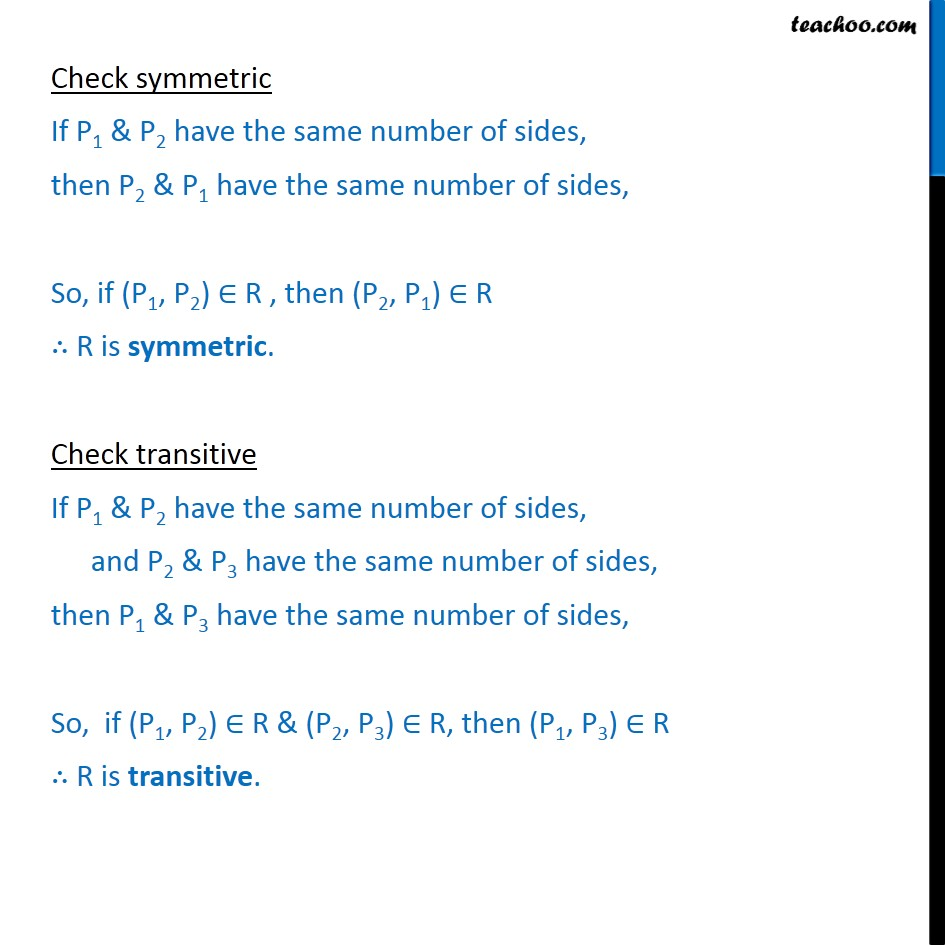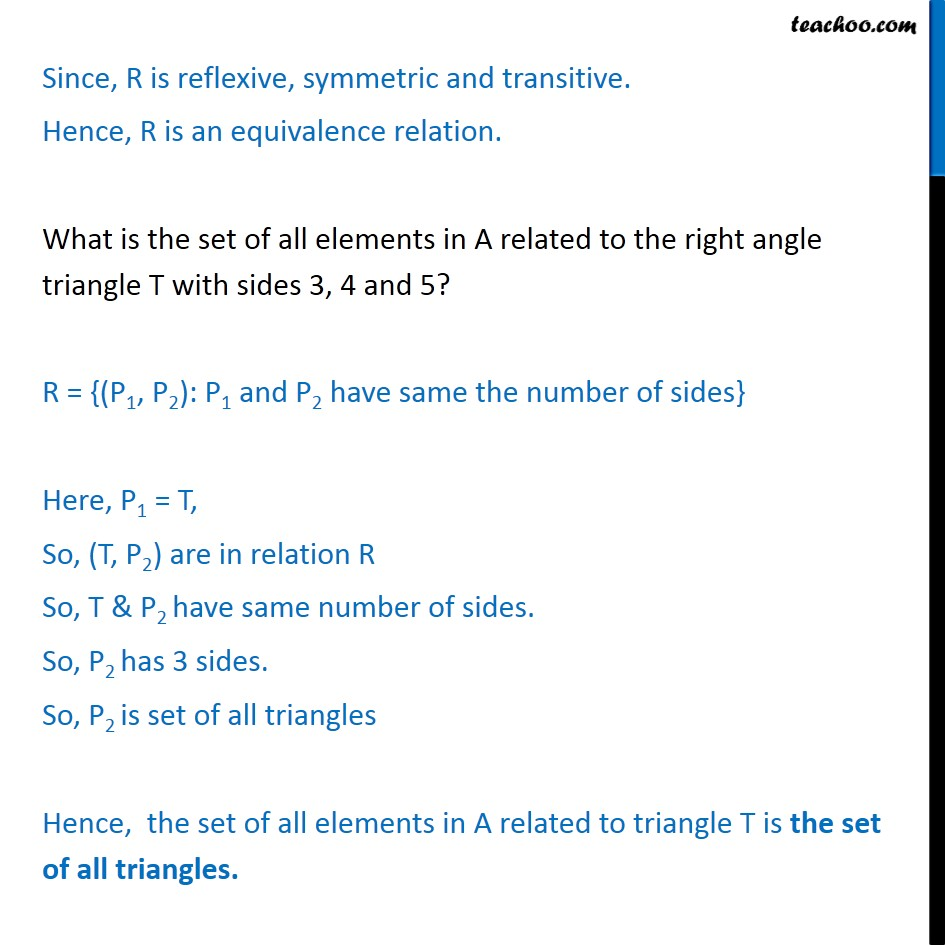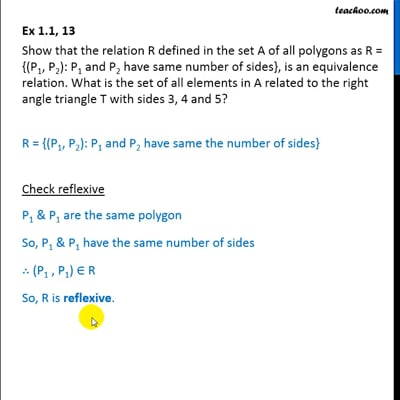To prove relation reflexive, transitive, symmetric and equivalent

Chapter 1 Class 12 Relation and Functions
Concept wiseThis video is only available for Teachoo black users

Introducing your new favourite teacher - Teachoo Black, at only ₹83 per month

### Transcript

Ex 1.1, 13 Show that the relation R defined in the set A of all polygons as R = {(P1, P2): P1 and P2 have same number of sides}, is an equivalence relation. What is the set of all elements in A related to the right angle triangle T with sides 3, 4 and 5? R = {(P1, P2): P1 and P2 have same the number of sides} Check reflexive P1 & P1 are the same polygon So, P1 & P1 have the same number of sides ∴ (P1 , P1) ∈ R So, R is reflexive. Check symmetric If P1 & P2 have the same number of sides, then P2 & P1 have the same number of sides, So, if (P1, P2) ∈ R , then (P2, P1) ∈ R ∴ R is symmetric. Check transitive If P1 & P2 have the same number of sides, and P2 & P3 have the same number of sides, then P1 & P3 have the same number of sides, So, if (P1, P2) ∈ R & (P2, P3) ∈ R, then (P1, P3) ∈ R ∴ R is transitive. Since, R is reflexive, symmetric and transitive. Hence, R is an equivalence relation. What is the set of all elements in A related to the right angle triangle T with sides 3, 4 and 5? R = {(P1, P2): P1 and P2 have same the number of sides} Here, P1 = T, So, (T, P2) are in relation R So, T & P2 have same number of sides. So, P2 has 3 sides. So, P2 is set of all triangles Hence, the set of all elements in A related to triangle T is the set of all triangles.